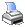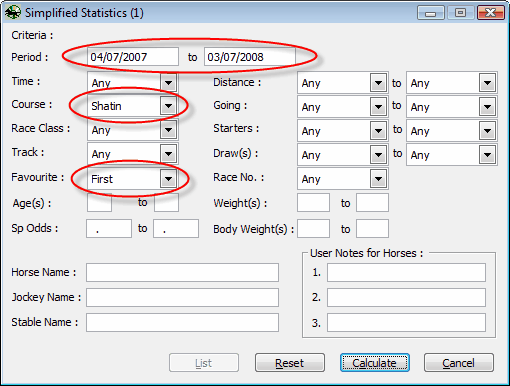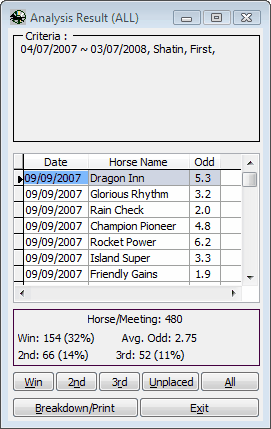﻿ Statistics and Data Analysis > Type 3: Simplified Statistics Form
 Navigation:  Statistics and Data Analysis > Type 3: Simplified Statistics FormSelect [Simplified Statistics] from the [Analysis] menu to open the Simplified Statistics form. Then set the criteria you want on the form and click on the [Calculate] button.The following form show the results.The above form shows that if we have betted \$10 in each race during the season 07-08, the total investment would then be \$4,800. We would have won 154 races. Since the average figure of the odds was 2.75, the return would then be \$4,235 and a lost \$565 (-13%) would have been got.

 Total Investment : 480 x \$10 = \$ 4,800.00 Return : 154 x 2.75 x \$10 = \$ 4,235.00 \$ -565.0 (-13%)